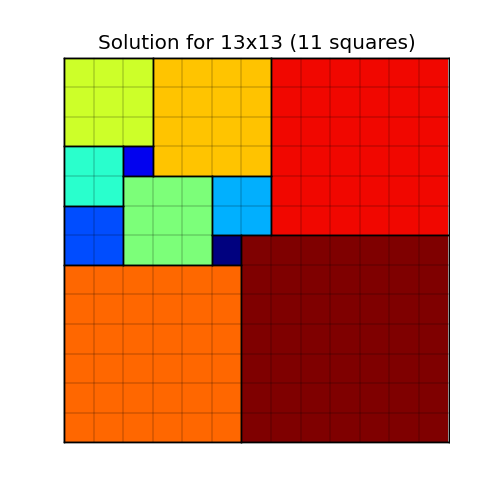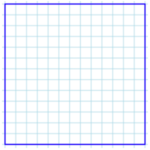# Squaring the squareThis Riddler puzzle is about tiling a square using smaller squares.

You are handed a piece of paper containing the 13-by-13 square shown below, and you must divide it into some smaller square pieces. If you are only allowed to cut along the lines, what is the smallest number of squares you can divide this larger square into? (You could, for example, divide it into one 12-by-12 square and 25 one-by-one squares for a total of 26 squares, but you can do much better.)Here is how I solved the problem:
[Show Solution]

And here is the tl;dr, just the solutions!
[Show Solution]

## 6 thoughts on “Squaring the square”

1.Tyler Barron says:

Very cool! Nice job

2.Stephen Meskin says:

Wow! Humbling: I thought this was an easy problem with 12 good for the 13×13 grid.
What does tl;dr mean?

1.Laurent says:

“too long; didn’t read”. For folks that want the answer right away without all the reading. 🙂

2.Hank says:

3.Clambo says:

You should consider marketing your solutions as a literal tile pattern for kitchens and bathrooms…some are quite pretty. Would be a niche clientele: nerds who are remodeling their houses.

4.Stephen Meskin says:

Conjecture:
Let p be the smallest prime number dividing n. For any number k let M(k) be the smallest number of squares > 1 that tile a k x k square, then M(n) = M(p).

Proof that M(n) <= M(p).
Consider a tiling of a p x p square with M(p) squares Multiply the length of each line segment by n/p. The result is a tiling of an n x n square by M(p) squares.

Can anyone provide a proof that M(p) <= M(n) or exhibit a counterexample?